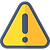### Cost Volume Profit

#### Margin of Safety

###### Content ContributorsPriya KaurChristian Bien

# Learning Objectives###### ​###### What is the Margin of Safety?The Margin of Safety refers to the amount of sales that are above the break-even point.

• High Margin of Safety: This indicates that the sales amount well exceeds the breakeven point. The Company has a relatively safe position at making a profit.

• Low Margin of Safety: This indicates that the sales amount is at or near the breakeven point. The Company is in a dangerous position as a slight decline in sales volumes could result in a loss.

What is safe and dangerous is dependent on the Company size and relative position to its competitors.

Calculation of Margin of Safety:

To Calculate the Margin of Safety in Units: Margin of Safety (Units) = Sales (Units) - Break-Even Point (Units)

To Calculate the Margin of Safety in Dollars: Margin of Safety Dollars = Sales (Dollars) - Breakeven (Dollars)

To calculate the Margin of Safety as a Percentage: Margin of Safety % = (Margin of Safety in Dollars) / (Actual/Budgeted Sales)###### Worked Example: Margin of SafetyWorked Example

MecBooks are a Computer Manufacturing Company. They sell their computers for a sale price of \$1000 and expect to sell 250 units this year.

The variable costs for each unit are \$800 and the fixed costs for the year is \$25,000.

Calculate the Margin of Safety in dollars and as a percentage.

Solution:

This question requires three separate parts, calculation of the contribution margin, calculation of the breakeven point and then a calculation of the margin of safety.

Step 1: Calculate the Contribution Margin

Recall from previous pages, that the contribution margin formula is as follows: Contribution Margin = Sales Price Per Unit - Variable Cost Per Unit The sale price is \$1000 in the question and variable costs are \$800. Therefore the Contribution Margin is: Contribution Margin = \$1000 - \$800 = \$200 per unit

Step 2: Calculate the Breakeven Point

Recall from previous pages, that the breakeven point formula is as follows:

Breakeven Point (Units) = Fixed Costs / Contribution Margin

The Fixed Costs are \$25,000 and the Contribution Margin has been calculated in Step 1 as \$200. Therefore the Breakeven Point is: Breakeven Point (Units) = \$25,000 / \$200 = 125 units

Step 3: Calculate the Margin of Safety

As stated in above, the Margin of Safety formula is as follows:

Margin of Safety = Sales (Units) - Break-Even Point (Units)

The expected sales is 250 units and the breakeven point is 125 units.

Therefore, the Margin of Safety is: Margin of Safety = 250 units - 125 units = 125 units

Hence, the Margin of Safety in Dollars is: 125 units * \$1,000 per unit sale price = \$125,000

To calculate the Margin of Safety as a Percentage: Margin of Safety % = (Margin of Safety in Dollars) / (Actual/Budgeted Sales) Budgeted sales was 250 units at \$1,000 each.

Hence Budgeted sales is 250 * \$1,000 = \$250,000. Hence Margin of Safety as a percentage is: Margin of Safety % = \$125,000 / \$250,000 Margin of Safety % = 50%###### ​###### ​###### ​###### ​###### ​###### ​